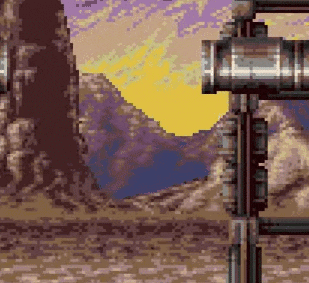# GMS 2Heat waves effect [SOLVED]

#### josyanf1

##### Member
Hello everyone! In arcade games I have seen that many use this technique of drawing waves with a sprite to give the sensation of heat / fire.Do you know what this effect is really called? Could you give me a hand to recreate it?

#### nacho_chicken

##### Member
The way retro games achieved this effect was a bit different, since they were drawing things pixel-by-pixel, but this effect should be relatively easy to do via shaders and the sine wave function sin(x).

Code:
``````varying vec2 v_vTexcoord;
varying vec4 v_vColour;

uniform float Time;
uniform vec2 Texel;

//Modify the following three consts to change the wave effect to your liking
const float xSpeed = 0.02;
const float xFreq = 75.0;
const float xSize = 1.5;

void main()
{
float xWave = sin(Time*xSpeed + v_vTexcoord.y*xFreq) * (xSize*Texel.x);
gl_FragColor = v_vColour * texture2D( gm_BaseTexture, v_vTexcoord + vec2(xWave, 0.0));
}``````
Object to draw waves with:
Code:
``````//Create event:

//Draw event:
var tex = sprite_get_texture(spr_background, 0);
draw_sprite_ext(spr_background, 0, x, y, xscale, yscale, 0, c_white, 1);

#### josyanf1

##### Member
The way retro games achieved this effect was a bit different, since they were drawing things pixel-by-pixel, but this effect should be relatively easy to do via shaders and the sine wave function sin(x).

Code:
``````varying vec2 v_vTexcoord;
varying vec4 v_vColour;

uniform float Time;
uniform vec2 Texel;

//Modify the following three consts to change the wave effect to your liking
const float xSpeed = 0.02;
const float xFreq = 75.0;
const float xSize = 1.5;

void main()
{
float xWave = sin(Time*xSpeed + v_vTexcoord.y*xFreq) * (xSize*Texel.x);
gl_FragColor = v_vColour * texture2D( gm_BaseTexture, v_vTexcoord + vec2(xWave, 0.0));
}``````
Object to draw waves with:
Code:
``````//Create event:
•